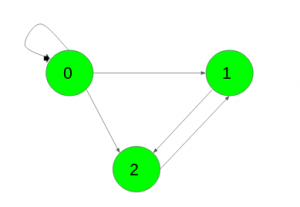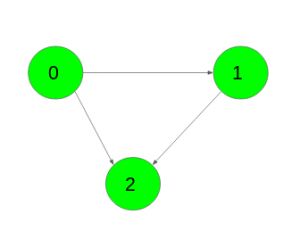# Find the number of paths of length K in a directed graph

Given a directed, unweighted graph with N vertices and an integer K. The task is to find the number of paths of length K for each pair of vertices (u, v). Paths don’t have to be simple i.e. vertices and edges can be visited any number of times in a single path.
The graph is represented as adjacency matrix where the value G[i][j] = 1 indicates that there is an edge from vertex i to vertex j and G[i][j] = 0 indicates no edge from i to j.

Examples:

Input: K = 2,Output:
1 2 2
0 1 0
0 0 1
Number of paths from 0 to 0 of length k is 1({0->0->0})
Number of paths from 0 to 1 of length k are 2({0->0->1}, {0->2->1})
Number of paths from 0 to 2 of length k are 2({0->0->2}, {0->1->2})
Number of paths from 1 to 1 of length k is 1({1->2->1})
Number of paths from 2 to 2 of length k is 1({2->1->2})

Input: K = 3,Output:
1 0 0
0 1 0
0 0 1
Number of paths from 0 to 0 of length k is 1({0->1->2->0})
Number of paths from 1 to 1 of length k is 1({1->2->0->1})
Number of paths from 2 to 2 of length k is 1({2->1->0->2})

## Recommended: Please try your approach on {IDE} first, before moving on to the solution.

Prerequisite: Matrix exponentiation, Matrix multiplication

Approach: It is obvious that given adjacency matrix is the answer to the problem for the case k = 1. It contains the number of paths of length 1 between each pair of vertices.
Let’s assume that the answer for some k is Matk and the answer for k + 1 is Matk + 1.

Matk + 1[i][j] = ∑p = 1NMatk[i][p]*G[p][j]

It is easy to see that the formula computes nothing other than the product of the matrices Matk and G i.e. Matk + 1 = Matk * G

Thus, the solution of the problem can be represented as Matk = G * G * … * G(k times) = Gk

Below is the implementation of the above approach:

## C++

 `// C++ implementation of the approach ` `#include ` `using` `namespace` `std; ` ` `  `#define N 3 ` ` `  `// Function to multiply two matrices ` `void` `multiply(``int` `a[][N], ``int` `b[][N], ``int` `res[][N]) ` `{ ` `    ``int` `mul[N][N]; ` `    ``for` `(``int` `i = 0; i < N; i++) { ` `        ``for` `(``int` `j = 0; j < N; j++) { ` `            ``mul[i][j] = 0; ` `            ``for` `(``int` `k = 0; k < N; k++) ` `                ``mul[i][j] += a[i][k] * b[k][j]; ` `        ``} ` `    ``} ` ` `  `    ``// Storing the multiplication result in res[][] ` `    ``for` `(``int` `i = 0; i < N; i++) ` `        ``for` `(``int` `j = 0; j < N; j++) ` `            ``res[i][j] = mul[i][j]; ` `} ` ` `  `// Function to compute G raised to the power n ` `void` `power(``int` `G[N][N], ``int` `res[N][N], ``int` `n) ` `{ ` ` `  `    ``// Base condition ` `    ``if` `(n == 1) { ` `        ``for` `(``int` `i = 0; i < N; i++) ` `            ``for` `(``int` `j = 0; j < N; j++) ` `                ``res[i][j] = G[i][j]; ` `        ``return``; ` `    ``} ` ` `  `    ``// Recursion call for first half ` `    ``power(G, res, n / 2); ` ` `  `    ``// Multiply two halves ` `    ``multiply(G, G, res); ` ` `  `    ``// If n is odd ` `    ``if` `(n % 2 != 0) ` `        ``multiply(res, G, res); ` `} ` ` `  `// Driver code ` `int` `main() ` `{ ` `    ``int` `G[N][N] = { { 1, 1, 1 }, ` `                    ``{ 0, 0, 1 }, ` `                    ``{ 0, 1, 0 } }; ` ` `  `    ``int` `k = 2, res[N][N]; ` ` `  `    ``power(G, res, k); ` ` `  `    ``for` `(``int` `i = 0; i < N; i++) { ` `        ``for` `(``int` `j = 0; j < N; j++) ` `            ``cout << res[i][j] << ``" "``; ` `        ``cout << ``"\n"``; ` `    ``} ` ` `  `    ``return` `0; ` `} `

## Java

 `// Java implementation of the approach ` `class` `GFG  ` `{ ` `     `  `static` `int` `N = ``3``; ` ` `  `// Function to multiply two matrices ` `static` `void` `multiply(``int` `a[][], ``int` `b[][], ``int` `res[][]) ` `{ ` `    ``int` `[][]mul = ``new` `int``[N][N]; ` `    ``for` `(``int` `i = ``0``; i < N; i++) ` `    ``{ ` `        ``for` `(``int` `j = ``0``; j < N; j++)  ` `        ``{ ` `            ``mul[i][j] = ``0``; ` `            ``for` `(``int` `k = ``0``; k < N; k++) ` `                ``mul[i][j] += a[i][k] * b[k][j]; ` `        ``} ` `    ``} ` ` `  `    ``// Storing the multiplication result in res[][] ` `    ``for` `(``int` `i = ``0``; i < N; i++) ` `        ``for` `(``int` `j = ``0``; j < N; j++) ` `            ``res[i][j] = mul[i][j]; ` `} ` ` `  `// Function to compute G raised to the power n ` `static` `void` `power(``int` `G[][], ``int` `res[][], ``int` `n) ` `{ ` ` `  `    ``// Base condition ` `    ``if` `(n == ``1``) { ` `        ``for` `(``int` `i = ``0``; i < N; i++) ` `            ``for` `(``int` `j = ``0``; j < N; j++) ` `                ``res[i][j] = G[i][j]; ` `        ``return``; ` `    ``} ` ` `  `    ``// Recursion call for first half ` `    ``power(G, res, n / ``2``); ` ` `  `    ``// Multiply two halves ` `    ``multiply(G, G, res); ` ` `  `    ``// If n is odd ` `    ``if` `(n % ``2` `!= ``0``) ` `        ``multiply(res, G, res); ` `} ` ` `  `// Driver code ` `public` `static` `void` `main(String[] args) ` `{ ` `    ``int` `G[][] = { { ``1``, ``1``, ``1` `}, ` `                    ``{ ``0``, ``0``, ``1` `}, ` `                    ``{ ``0``, ``1``, ``0` `} }; ` ` `  `    ``int` `k = ``2``; ` `    ``int` `[][]res = ``new` `int``[N][N]; ` ` `  `    ``power(G, res, k); ` ` `  `    ``for` `(``int` `i = ``0``; i < N; i++)  ` `    ``{ ` `        ``for` `(``int` `j = ``0``; j < N; j++) ` `            ``System.out.print(res[i][j] + ``" "``); ` `        ``System.out.println(``""``); ` `    ``} ` `} ` `} ` ` `  `// This code is contributed by 29AjayKumar `

## Python3

 `# Python3 implementation of the approach  ` ` `  `import` `numpy as np ` ` `  `N ``=` `3`  ` `  `# Function to multiply two matrices  ` `def` `multiply(a, b, res) :  ` ` `  `    ``mul ``=` `np.zeros((N,N));  ` `     `  `    ``for` `i ``in` `range``(N) : ` `        ``for` `j ``in` `range``(N) : ` `            ``mul[i][j] ``=` `0``;  ` `            ``for` `k ``in` `range``(N) :  ` `                ``mul[i][j] ``+``=` `a[i][k] ``*` `b[k][j];  ` ` `  `    ``# Storing the multiplication result in res[][]  ` `    ``for` `i ``in` `range``(N) : ` `        ``for` `j ``in` `range``(N) : ` `            ``res[i][j] ``=` `mul[i][j];  ` ` `  ` `  `# Function to compute G raised to the power n  ` `def` `power(G, res, n) : ` `     `  `    ``# Base condition  ` `    ``if` `(n ``=``=` `1``) : ` `        ``for` `i ``in` `range``(N) :  ` `            ``for` `j ``in` `range``(N) : ` `                ``res[i][j] ``=` `G[i][j];  ` `        ``return``; ` ` `  `    ``# Recursion call for first half  ` `    ``power(G, res, n ``/``/` `2``);  ` ` `  `    ``# Multiply two halves  ` `    ``multiply(G, G, res);  ` ` `  `    ``# If n is odd  ` `    ``if` `(n ``%` `2` `!``=` `0``) : ` `        ``multiply(res, G, res);  ` ` `  `# Driver code  ` `if` `__name__ ``=``=` `"__main__"` `:  ` ` `  `    ``G ``=` `[  ` `        ``[ ``1``, ``1``, ``1` `],  ` `        ``[ ``0``, ``0``, ``1` `],  ` `        ``[ ``0``, ``1``, ``0` `]  ` `        ``];  ` ` `  `    ``k ``=` `2``;  ` `    ``res ``=` `np.zeros((N,N));  ` ` `  `    ``power(G, res, k);  ` ` `  `    ``for` `i ``in` `range``(N) : ` `        ``for` `j ``in` `range``(N) : ` `            ``print``(res[i][j],end ``=` `" "``); ` `         `  `        ``print``()  ` `         `  `# This code is contributed by AnkitRai01 `

## C#

 `// C# implementation of the approach ` `using` `System; ` ` `  `class` `GFG  ` `{ ` `     `  `static` `int` `N = 3; ` ` `  `// Function to multiply two matrices ` `static` `void` `multiply(``int` `[,]a, ``int` `[,]b, ``int` `[,]res) ` `{ ` `    ``int` `[,]mul = ``new` `int``[N,N]; ` `    ``for` `(``int` `i = 0; i < N; i++) ` `    ``{ ` `        ``for` `(``int` `j = 0; j < N; j++)  ` `        ``{ ` `            ``mul[i,j] = 0; ` `            ``for` `(``int` `k = 0; k < N; k++) ` `                ``mul[i,j] += a[i,k] * b[k,j]; ` `        ``} ` `    ``} ` ` `  `    ``// Storing the multiplication result in res[][] ` `    ``for` `(``int` `i = 0; i < N; i++) ` `        ``for` `(``int` `j = 0; j < N; j++) ` `            ``res[i,j] = mul[i,j]; ` `} ` ` `  `// Function to compute G raised to the power n ` `static` `void` `power(``int` `[,]G, ``int` `[,]res, ``int` `n) ` `{ ` ` `  `    ``// Base condition ` `    ``if` `(n == 1) { ` `        ``for` `(``int` `i = 0; i < N; i++) ` `            ``for` `(``int` `j = 0; j < N; j++) ` `                ``res[i,j] = G[i,j]; ` `        ``return``; ` `    ``} ` ` `  `    ``// Recursion call for first half ` `    ``power(G, res, n / 2); ` ` `  `    ``// Multiply two halves ` `    ``multiply(G, G, res); ` ` `  `    ``// If n is odd ` `    ``if` `(n % 2 != 0) ` `        ``multiply(res, G, res); ` `} ` ` `  `// Driver code ` `public` `static` `void` `Main() ` `{ ` `    ``int` `[,]G = { { 1, 1, 1 }, ` `                    ``{ 0, 0, 1 }, ` `                    ``{ 0, 1, 0 } }; ` ` `  `    ``int` `k = 2; ` `    ``int` `[,]res = ``new` `int``[N,N]; ` ` `  `    ``power(G, res, k); ` ` `  `    ``for` `(``int` `i = 0; i < N; i++)  ` `    ``{ ` `        ``for` `(``int` `j = 0; j < N; j++) ` `            ``Console.Write(res[i,j] + ``" "``); ` `        ``Console.WriteLine(``""``); ` `    ``} ` `} ` `} ` ` `  `// This code is contributed by anuj_67.. `

Output:

```1 2 2
0 1 0
0 0 1
```

My Personal Notes arrow_drop_uppawanasipugmailcom

If you like GeeksforGeeks and would like to contribute, you can also write an article using contribute.geeksforgeeks.org or mail your article to contribute@geeksforgeeks.org. See your article appearing on the GeeksforGeeks main page and help other Geeks.

Please Improve this article if you find anything incorrect by clicking on the "Improve Article" button below.

Improved By : 29AjayKumar, vt_m, AnkitRai01

Article Tags :
Practice Tags :

Be the First to upvote.

Please write to us at contribute@geeksforgeeks.org to report any issue with the above content.